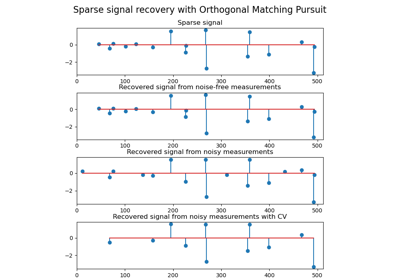# sklearn.linear_model.OrthogonalMatchingPursuit¶

class sklearn.linear_model.OrthogonalMatchingPursuit(*, n_nonzero_coefs=None, tol=None, fit_intercept=True, normalize=True, precompute='auto')[source]

Orthogonal Matching Pursuit model (OMP)

Read more in the User Guide.

Parameters
n_nonzero_coefsint, default=None

Desired number of non-zero entries in the solution. If None (by default) this value is set to 10% of n_features.

tolfloat, default=None

Maximum norm of the residual. If not None, overrides n_nonzero_coefs.

fit_interceptboolean, default=True

whether to calculate the intercept for this model. If set to false, no intercept will be used in calculations (i.e. data is expected to be centered).

normalizeboolean, default=True

This parameter is ignored when fit_intercept is set to False. If True, the regressors X will be normalized before regression by subtracting the mean and dividing by the l2-norm. If you wish to standardize, please use StandardScaler before calling fit on an estimator with normalize=False.

precompute{True, False, ‘auto’}, default=’auto’

Whether to use a precomputed Gram and Xy matrix to speed up calculations. Improves performance when n_targets or n_samples is very large. Note that if you already have such matrices, you can pass them directly to the fit method.

Attributes
coef_array, shape (n_features,) or (n_targets, n_features)

parameter vector (w in the formula)

intercept_float or array, shape (n_targets,)

independent term in decision function.

n_iter_int or array-like

Number of active features across every target.

Notes

Orthogonal matching pursuit was introduced in G. Mallat, Z. Zhang, Matching pursuits with time-frequency dictionaries, IEEE Transactions on Signal Processing, Vol. 41, No. 12. (December 1993), pp. 3397-3415. (http://blanche.polytechnique.fr/~mallat/papiers/MallatPursuit93.pdf)

This implementation is based on Rubinstein, R., Zibulevsky, M. and Elad, M., Efficient Implementation of the K-SVD Algorithm using Batch Orthogonal Matching Pursuit Technical Report - CS Technion, April 2008. https://www.cs.technion.ac.il/~ronrubin/Publications/KSVD-OMP-v2.pdf

Examples

>>> from sklearn.linear_model import OrthogonalMatchingPursuit
>>> from sklearn.datasets import make_regression
>>> X, y = make_regression(noise=4, random_state=0)
>>> reg = OrthogonalMatchingPursuit().fit(X, y)
>>> reg.score(X, y)
0.9991...
>>> reg.predict(X[:1,])
array([-78.3854...])


Methods

 fit(X, y) Fit the model using X, y as training data. get_params([deep]) Get parameters for this estimator. Predict using the linear model. score(X, y[, sample_weight]) Return the coefficient of determination R^2 of the prediction. set_params(**params) Set the parameters of this estimator.
fit(X, y)[source]

Fit the model using X, y as training data.

Parameters
Xarray-like, shape (n_samples, n_features)

Training data.

yarray-like, shape (n_samples,) or (n_samples, n_targets)

Target values. Will be cast to X’s dtype if necessary

Returns
selfobject

returns an instance of self.

get_params(deep=True)[source]

Get parameters for this estimator.

Parameters
deepbool, default=True

If True, will return the parameters for this estimator and contained subobjects that are estimators.

Returns
paramsmapping of string to any

Parameter names mapped to their values.

predict(X)[source]

Predict using the linear model.

Parameters
Xarray-like or sparse matrix, shape (n_samples, n_features)

Samples.

Returns
Carray, shape (n_samples,)

Returns predicted values.

score(X, y, sample_weight=None)[source]

Return the coefficient of determination R^2 of the prediction.

The coefficient R^2 is defined as (1 - u/v), where u is the residual sum of squares ((y_true - y_pred) ** 2).sum() and v is the total sum of squares ((y_true - y_true.mean()) ** 2).sum(). The best possible score is 1.0 and it can be negative (because the model can be arbitrarily worse). A constant model that always predicts the expected value of y, disregarding the input features, would get a R^2 score of 0.0.

Parameters
Xarray-like of shape (n_samples, n_features)

Test samples. For some estimators this may be a precomputed kernel matrix or a list of generic objects instead, shape = (n_samples, n_samples_fitted), where n_samples_fitted is the number of samples used in the fitting for the estimator.

yarray-like of shape (n_samples,) or (n_samples, n_outputs)

True values for X.

sample_weightarray-like of shape (n_samples,), default=None

Sample weights.

Returns
scorefloat

R^2 of self.predict(X) wrt. y.

Notes

The R2 score used when calling score on a regressor uses multioutput='uniform_average' from version 0.23 to keep consistent with default value of r2_score. This influences the score method of all the multioutput regressors (except for MultiOutputRegressor).

set_params(**params)[source]

Set the parameters of this estimator.

The method works on simple estimators as well as on nested objects (such as pipelines). The latter have parameters of the form <component>__<parameter> so that it’s possible to update each component of a nested object.

Parameters
**paramsdict

Estimator parameters.

Returns
selfobject

Estimator instance.

## Examples using sklearn.linear_model.OrthogonalMatchingPursuit¶